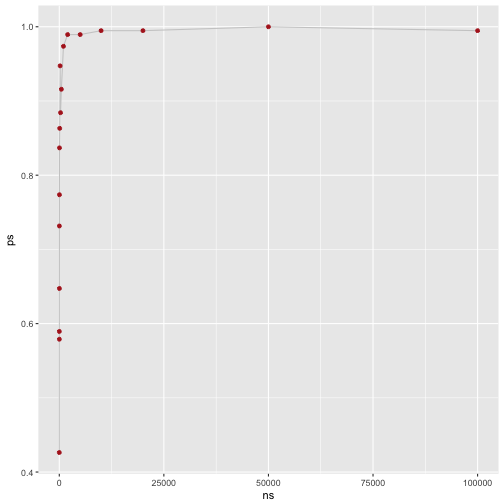# The effect of sample on p-values. A simulation.

It is well-known that the notorious p-values is sensitive to sample size: The larger the sample, the more bound the p-value is to fall below the magic number of .05.

Of course, the p-value is also a function of the effect size, eg., the distance between two means and the respective variances. But still, the p-values tends to become significant in the face of larges samples, and non-significant otherwise.

Theoretically, quite simple and well understood. But let’s take the test of “real” data and do a simulation to demonstrate or test this behavior.

library(tidyverse)


# Simulate data with large sample size

Next, we simulate data: A data frame of 20 cols and many rows (1e06, ie., 1 million). We should also make sure that the null hypothesis is false in our data. To that end, we let the mean values of the columns vary somewhat.

k <- 20
n <- 1e06
df <- data.frame(replicate(k,rnorm(n = n, mean = rnorm(1, 0, 1), sd = 1)))


Now let’s compute t-tests for each and every combination (cartesian product of all combinations). We will save the resulting p-values in a (square) matrix.

m <- matrix(nrow = k, ncol = k)

for (i in seq_len(ncol(df))) {
for (j in seq_len(ncol(df))) {
m[i, j] <- t.test(df[i], df[j])$p.value } }  One half of the matrix is redundant, as the matrix is symmetric. The same reasoning applies for the diagonal. Let’s take out the redundant elements. m[lower.tri(m)] <- NA m[diag(m)] <- NA  Let’s come up with a logical matrix indicating whether one cell (ie., one t-test) indicates a significant t-test (TRUE) or not (FALSE). m_significant <- apply(m, c(1,2), function(x) x < .05)  Finally, let’s count the number of significant results, and sum then up. m_significant %>% sum(TRUE, na.rm = TRUE)  ##  191  The number of different tests is $$(k*k - k)/2$$. Which amounts, in this case to (k*k-20)/2  ##  190  Hence, all tests are significant. rm(df)  # Simulate data with small sample size Now, we repeat the same thing with a small sample. simulate_ps <- function(n = 1e06, k = 20){ # arguments: # n: number of rows # k: number of columns # returns: proportion of significant (p<.05) t-tests set.seed(42) # simulate data df <- data.frame(replicate(k,rnorm(n = n, mean = rnorm(1, 0, 1), sd = 1))) # matrix for t-test results m <- matrix(nrow = k, ncol = k) # cartesian product of all t-tests for (i in seq_len(ncol(df))) { for (j in seq_len(ncol(df))) { m[i, j] <- t.test(df[i], df[j])$p.value
}
}

# take-out redundant cells
m[lower.tri(m)] <- NA
m[diag(m)] <- NA

# compute matrix to count number of significant t-tests
m_significant <- apply(m, c(1,2), function(x) x < .05)

# count
sum_significant <- m_significant %>% sum(TRUE, na.rm = TRUE)

sum_distinct_tests <- (k*k - k)/2

prop_significant <- sum_significant / sum_distinct_tests

rm(df)
return(prop_significant)

}

simulate_ps(n = 10, k = 20)

##  0.5894737


# Play around

Now, we can play around a bit.

ns <- c(5, 10, 15, 20, 30, 40, 50, 100, 200, 300, 500, 1000, 2000, 5000, 10000, 2e04, 5e04, 1e05)

ps <- vector(mode = "numeric", length = length(ns))

for (i in seq_along(ns)){
ps[i] <- simulate_ps(n = ns[i], k = 20)
print(ps[i])
}

##  0.4263158
##  0.5894737
##  0.5789474
##  0.7315789
##  0.6473684
##  0.7736842
##  0.8368421
##  0.8631579
##  0.9473684
##  0.8842105
##  0.9157895
##  0.9736842
##  0.9894737
##  0.9894737
##  0.9947368
##  0.9947368
##  1
##  0.9947368


Finally, let’s plot that:

data_frame(
ns = ns,
ps = ps
)  %>%
ggplot +
aes(x = ns, y = ps) +
geom_line(color = "gray80") +
geom_point(color = "firebrick")Thus, our result appears reasonable: The larger the sample size (ns), the higher the proportion of ps (ps).